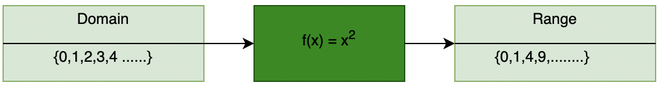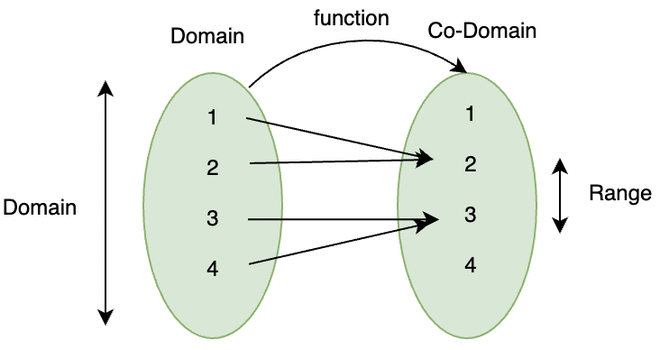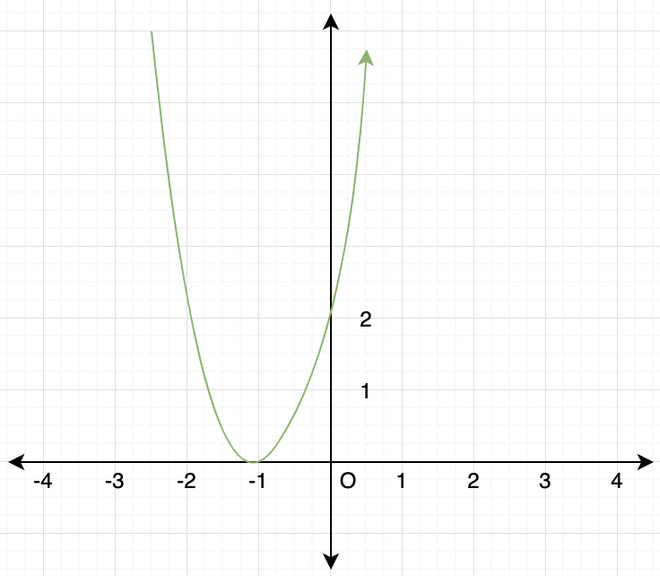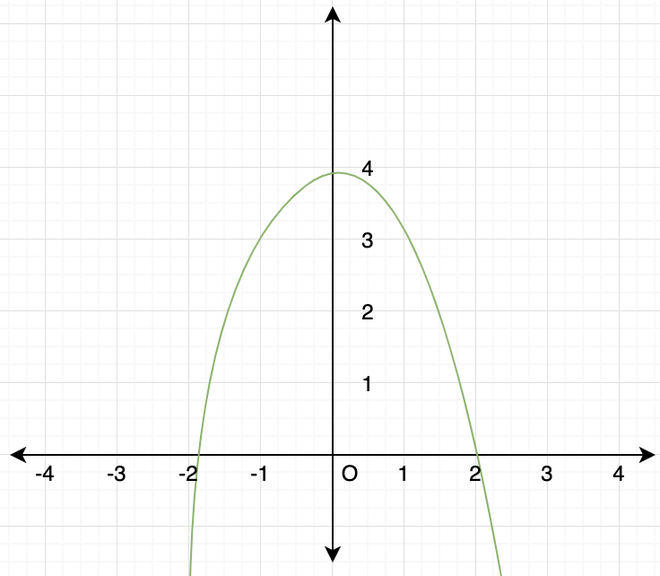Open In App
Related Articles
• CBSE Class 11 Maths Notes
• CBSE Class 11 Maths Formulas
• NCERT Solutions for Class 11 Maths
• RD Sharma Class 11 Solutions for Maths

# Range of a Function

Functions in math can be thought of as vending machines. Given the money in the form of input, they give some cans or cookies in return. Similarly, functions take some input numbers and give us some output. It can be said that, in real life, Everything can be formulated and solved with the help of functions. From building design and Architecture to Mega Skyscrapers, the mathematical model of almost everything in real life requires Functions, Therefore, it can’t be avoided that functions have gigantic significance in our lives. Domain and Range are one aspect through which a function can be described.

For example: Suppose it’s written on top of the machine that only Rs.20 and Rs.50 notes can be used to buy something. What if, someone uses Rs.10 notes? The machine will not give any output. So, the domain represents what kind of inputs we can have in a function. In this case, Rs.20 and Rs.50 notes are the domain of the “Vending Machine”. Similarly, it doesn’t matter how much money one puts in the machine, he/she will never get Sandwiches from it. So, the concept of the range comes into play here, range is the possible outputs machine can give.

### Range and Domain of a Function

Domain of a Function:

A domain is all the values that can go into a function for which it gives a valid output. It is the set of all the possible inputs to a function.

For example: In the figure below, f(x) = x2. The set of all the inputs is called Domain and the set of all the outputs is considered as the range.How to find the domain of a function?

The domain of the function should contain all real numbers except the points where the denominator becomes zero and terms under square roots become negative. To find the domain, try to find the points or input values over which the function is not defined.

Question 1: Find the domain ofThis function can give undefined output when x = 1. So, then domain is R – {1}

Question 2: Find the Domain of the following function:It is Important to not make the function either Infinity or Undefined, therefore, we need to see what Domain values can make the Function Undefined or Infinity.

Taking a look at the denominator, It is clear that the values 3 and 5 are making the denominator 0, hence, making the function Infinite which is not desirable.

Therefore, the values x=3 and x=5 can’t be placed here.

The Domain will be R – {3,5}.

Question 3: Find the Domain values for which the Functions Y = (2x2-1) and Z= (1-3x) are equal.

Equating the two Functions:

2 x2– 1 = 1 – 3 x

2x2 + 3x – 2 = 0

2x2 + 4x – x – 2 = 0

2x (x + 2) – 1 (x+2)= 0

(2x – 1) (x + 2) = 0

x = 1/2, -2.

Therefore, the Domain values are {1/2, -2}.

### Range of a Function

The range of a function is a set of all its possible outputs.

Example: Let’s consider a function ƒ: A⇢A, where A = {1,2,3,4}.The elements of the set Domain, are called pre-images, and elements of the set Co-Domain which are mapped to pre-images are called images. The range of a function is a set of all the images of elements in the domain. In this example, the range of the function is {2,3}.

How to find the range of a function?

The range is the spread of the values of the output of a function. If we are able to calculate the maximum and the minimum values of the function, we can get an idea of the range of the function.

Question 1: Find the range. f(x) =Now, since the function is a square root, it can never give negative values as output. So, the minimum value can only be 0 at x = 1. Maximum value can go up to infinity as we keep on increasing x.

So, the range of the function is [0,∞).

Question 2: The domain of the function ƒ defined by f(x) =is?

Given, f(x) =One has to ensure two things while selecting the set of domain,

• Denominator never goes to zero.
• Term is inside the square root doesn’t become negative.

Let’s expand what’s written inside term within square root.In this case, we cannot put either of the values, x ≥ 0 or x < 0.

Hence, f is not defined for any x ∈ R. So, the domain is an empty set.

### Domain and Range of Quadratic Functions

Quadratic functions are the functions of the form f(x) = ax2 + bx + c, where a, b and c are constants and a ≠ 0.  The graph of a quadratic function is in the form of a parabola. It is basically a curved shape opening up or down.

Let’s look at how to graph quadratic functions,

• if a > 0, the parabola opens upwards.
• if a < 0, the parabola opens downwards.

Now, the vertex is the highest or lowest point of our curve depending on the graph of the quadratic function. To find the vertex of the graph of a general quadratic expression.

In the standard quadratic form, the vertex is given byOne has to find the x-value of the vertex first and then just has to plug it in into the function to get the y-value.

Note: Each curve is symmetrical around its vertical axis.

Let’s look at some examples,

Question: Plot the graph of f(x) = 2x2 + 4x + 2.

Comparing this equation with the general quadratic function equation. a = 2, b = -4 and c = 2.

Since a > 0, this parabola will open upwards.

• Vertex x-value =• Vertex y-value = 2(-1)2 + 4(-1) + 2 = 0

So, the vertex is at (-1,0). Since the parabola opens upwards, this must be the minimum value of the function.

The point where graph cuts y-axis is (0,2).Range and Domain of quadratic functions can be found out easily by plotting the graph. It’s not always necessary to plot the full graph, for range only the direction of the parabola(upward or downward) and value of the parabola at vertex should be known. Value at the vertex is always either minimum/maximum depending upon the direction of the parabola. The domain of such functions is always whole real numbers because there are defined everywhere i.e; there is no value of input which might cause them to give undefined as output.

Let’s look at another example regarding the domain and range of the parabola.

Question: Plot the graph and find domain and range of the given function, f(x) = -x2 + 4.